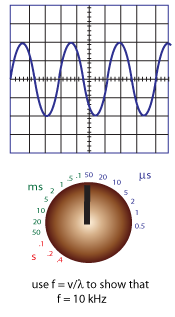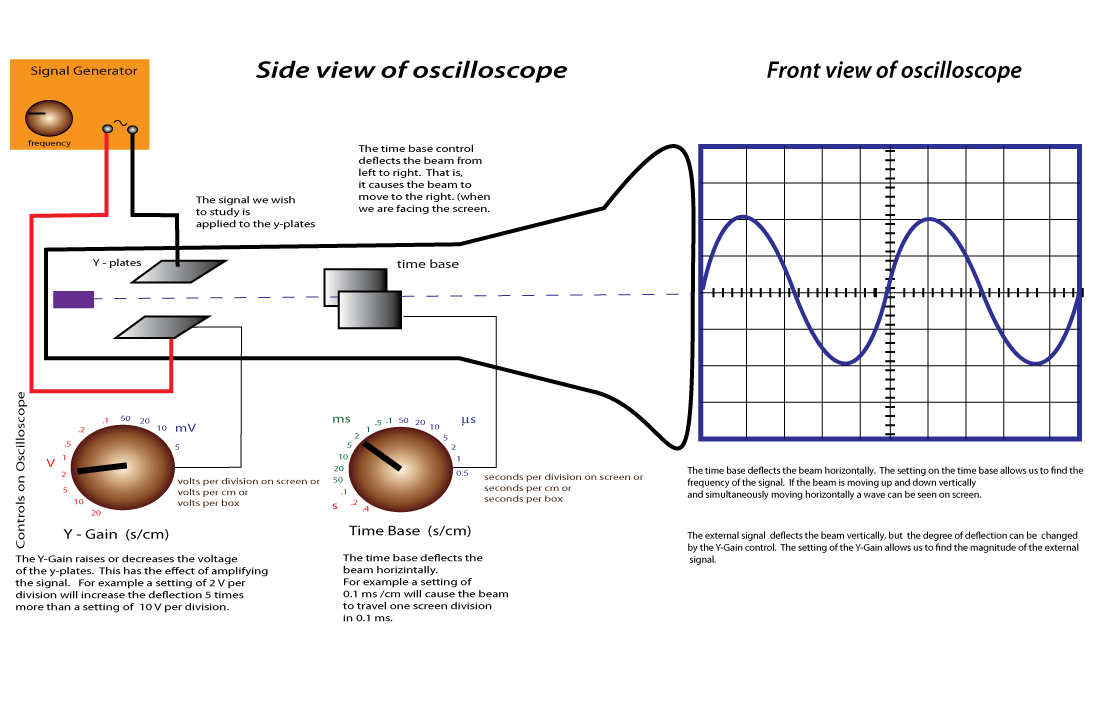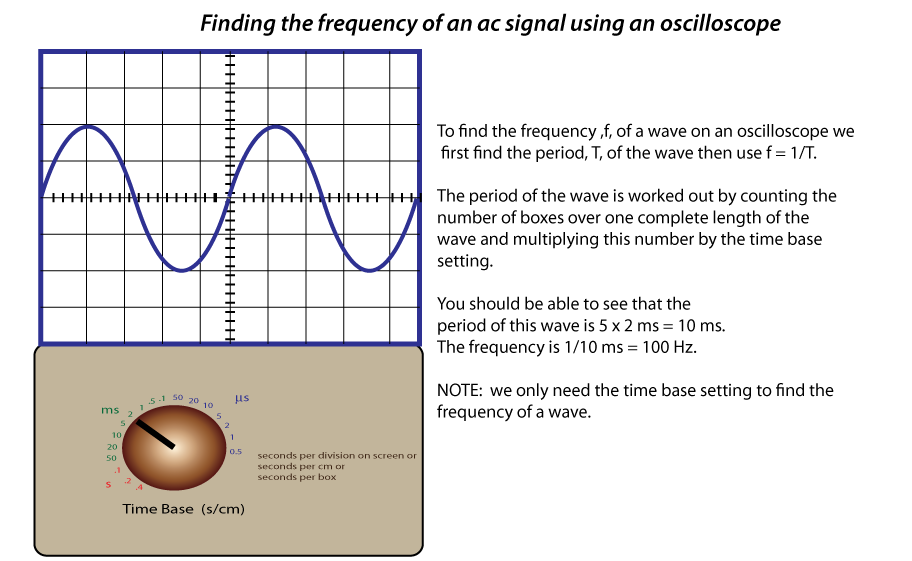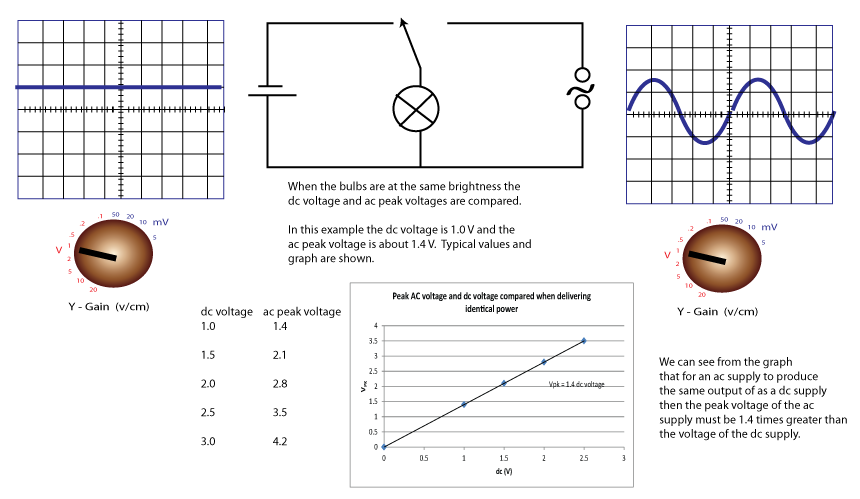## Learning Outcomes

Aids to Understanding

The Oscilloscope

An oscilloscope is a device for visually analysing electrical signals. The signals can be either dc or ac. When we switch on an oscilloscope a beam of negatively charged particles flow from the back of the oscilloscope tube to the screen at the front. This beam strikes the screen and causes it to glow. The colour the screen glows depends on the fluorescent colouring of the screen. The signal we wish to analyse deflects the beam in the vertical direction, only. The engineer observes and measures this deflection and thus learns information about the signal he/she wishes to analyze.

Finding the frequency of an ac signal

The main body text discusses one method of find the frequency of an ac signal. An alternative method uses f = v/l.
The wavelength can be found counting the number of horizontal boxes that take up one complete wavelength and the speed can be found using d/t where d is the wavelength and t is the period. Verify the wave in the diagram below has a frequency of 10 kHz.Alternating Signals

Whenever we plug in a bulb to the mains the device is connected to a 50 Hz, 325 Vpeak signal. Thus the bulb recieves a signal that is continuously changing in magnitude that reverses direction every 0.01 s. The maximum signal or peak signal, the bulb receives is 325 V and the minimum is 0 V. As we know the signal can be represented as a wave form. That the bulb's brightness seems to be unchanging makes that statement seem odd, but if the frequency of the signal is low enough then we can clearly see the flashing bulb.

The root mean square voltage of a mains signal.

If we wish to find the power developed in a bulb connected to a 6.0 V dc supply with current of 3.0 A then we use P = IV = 18 W. We use the same expression to find the power developed in a bulb connected to an ac supply. Our problem is choosing what I and V to use. Clearly we cannot use the peak current and voltage because the bulb only receives the peak signal every half cycle, or 0.01 s if we are considering the mains signal. To find the effective power output of the bulb We use the root mean square voltage of the signal which is given by Vrms = Vpk ⁄ √ 2. The effective power output of the bulb is given by Peff = VrmsIrms, where Irms = Ipk ⁄ √ 2

## 1) Using the oscilloscope to measuring the frequency of a signal

The diagram below shows the essential internal parts of an oscilloscope. The electron beam travels from the back of the oscilloscope (far left) to the screen. The two sets of deflection plates deflect the beam vertically and horizontally. Study the diagram below and try to understand the basic working of this device before we use it to find the frequency of electrical signals.## 2) The root mean square voltage of an ac signal

If we connect a bulb to a low frequency ac signal then the bulb will be seen to continuously change in brightness. These changes in brightness are not seen if the frequency of the signal is sufficiently high.If we wish to find the power developed in a bulb connected to a 6.0 V dc supply with current of 3.0 A then we use P = IV = 18 W. We use the same expression to find the power developed in a bulb connected to an ac supply. Our problem is choosing what I and V to use. Clearly we cannot use the peak current and voltage because the bulb only receives the peak signal every half cycle, or 0.01 s if we are considering the mains signal. To solve this problem we get a bulb and connect it into a dc supply and observe its brightness (power output). We connect the same bulb into an ac supply and adjust the amplitude until the bulb is at the same brightness as it was with the dc supply. We do this for a few different dc voltages. We then compare the peak voltages of the ac signal with the actual voltage of the dc supplies and look for a pattern. The set up below illustrates this procedure.A mathematical analysis shows the multiplicative factor is not 1.4 but precisely √ 2. From this analysis we define the root mean square voltage, Vrms, of an ac signal with peak voltage, Vpk, as Vpk/√ 2. The Vrms voltage of an ac signal is equal to the dc voltage that will deliver the same power output.Example> If the peak signal of the mains is 322 V and it is delivering current to a bulb with resistance 1000 W, then show that the effective power output of the bulb is about 53 W.